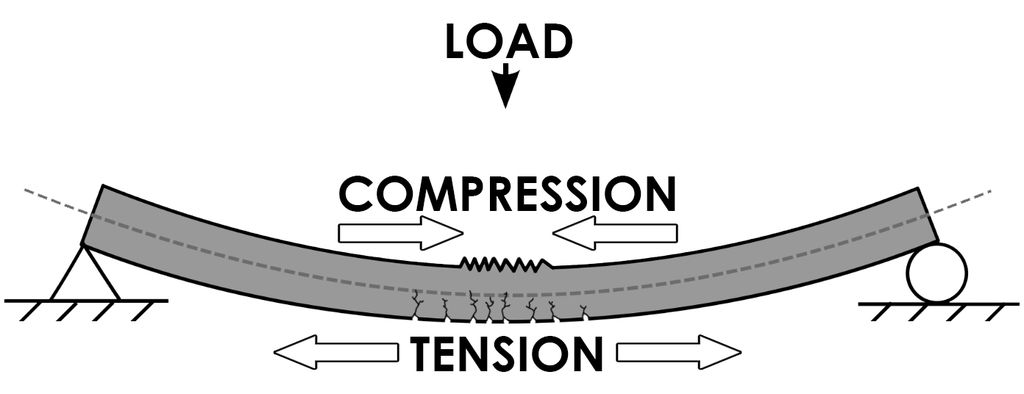## Concrete in Tension: Tensile Strength of Concrete### Concrete in Tension: Tensile Strength of Concrete

The tensile and compressive strengths of concrete are not proportional, and an increase in compressive strength is accompanied by an appreciably smaller percentage increase in tensile strength. According to the ACI Code Commentary, the tensile strength of normal-weight concrete in flexure is about 10% to 15% of the compressive strength.

The true tensile strength of concrete is difficult to determine. The split-cylinder test (ASTM C496) has been  used to determine the tensile strength of lightweight aggregate concrete and is generally accepted as a good measure of the true tensile strength. The split-cylinder test uses a standard 6-in.-diameter, 12-in.-long cylinder placed on its side in a testing machine. A compressive line load is applied uniformly along the length of the cylinder, with support furnished along the full length of the bottom of the cylinder. The compressive load produces a transverse tensile stress, and the cylinder will split in half along a diameter when its tensile strength is reached.

The tensile stress at which splitting occurs is referred to as the splitting tensile strength, fct, and may be calculated by the following expression derived from the theory of elasticity:where

fct = splitting tensile strength of lightweight aggregate concrete (psi)
P = applied load at splitting (lb)
L = length of cylinder (in.)
D = diameter of cylinder (in.)

Another common approach has been to use the modulus of rupture, fr (which is the maximum tensile bending stress in a plain concrete test beam at failure), as a measure of tensile strength (ASTM C78). The moment that produces a tensile stress just equal to the modulus of rupture is termed the cracking moment, Mcr

The ACI Code recommends that the modulus of rupture fr be taken as, where fcis in psi, λ is a modification factor reflecting the lower tensile strength of lightweight concrete relative to normal-weight concrete.

The values for λ are as follows:

Normal-weight concrete—1.0
Sand-lightweight concrete—0.85
All-lightweight concrete—0.75

Interpolation between these values is permitted.

If the average splitting tensile strength fct is specified, then